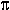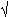DOC HOME SITE MAP MAN PAGES GNU INFO SEARCH PRINT BOOK

# math(5)

math -- math functions and constants

## Synopsis

```   #include <math.h>
```

## Description

This file contains declarations of all the functions in the Math Library (described in Section 3M), as well as various functions in the C Library (Section 3C) that return double, float and long double-precision values.

It defines the structure and constants used by the matherr(3M) error-handling mechanisms, including the following constant used as an error-return value:

HUGE
The maximum value of a single-precision floating-point number.

The following mathematical constants are defined for user convenience:

M_E
The base of natural logarithms (e).

M_LOG2E
The base-2 logarithm of e.

M_LOG10E
The base-10 logarithm of e.

M_LN2
The natural logarithm of 2.

M_LN10
The natural logarithm of 10.

M_PI, the ratio of the circumference of a circle to its diameter.

M_PI_2/2.

M_PI_4/4.

M_1_PI
1/.

M_2_PI
2/.

M_2_SQRTPI
2/.

M_SQRT2
The positive square root of 2.

M_SQRT1_2
The positive square root of 1/2.

The numerical classification constants are defined in this header file:

FP_INFINITE

FP_NAN

FP_NORMAL

FP_SUBNORMAL

FP_ZERO
represent the mutually exclusive kinds of possible floating-point values. They expand to integer constant expressions with distinct values.

The following fma performance indicator constants are defined in this header file:

FP_FAST_FMA
If defined, FP_FAST_FMA indicates that fma(3M) generally executes about as fast as, or faster than a multiply and an add of double operands.

FP_FAST_FMAF
If defined, FP_FAST_FMF indicates that fmaf(3M) generally executes about as fast as, or faster than a multiply and an add of float operands.

FP_FAST_FMAL
If defined, FP_FAST_FMAL indicates that fmal(3M) generally executes about as fast as, or faster than a multiply and an add of long double operands.

NOTE: Typically, these constants are only defined if the fma functions are implemented directly with a hardware multiply-add instruction.

The following ilogb constants are defined in this header file:

FP_ILOGB0
Is set to either INT_MIN or -INT_MAX when ilogb(3M) is called with its argument set to zero.

FP_ILOGBNAN
Is set to either INT_MAX or INT_MIN when ilogb(3M) is called with its argument set to NaN.

The following integer constants used by math_errhandling are defined in this header file:

MATH_ERRNO
Expands to 1.

MATH_ERREXCEPT
Expands to 2.

The following mathematical constants are also defined in this header file:

MAXFLOAT
The maximum value of a non-infinite single-precision floating point number.

HUGE_VAL
A double constant expression representing positive infinity.

HUGE_VALF
A float constant expression representing positive infinity.

HUGE_VALL
A long double constant expression representing positive infinity.

The following constant is only defined on machines that support quiet NaNs for the float type:

NAN
A float constant expression representing a quiet NaN.

For the definitions of various machine-dependent constants, see values(5).

## References

intro(3), matherr(3M), fma(3M), fmaf(3M), fmal(3M), ilogb(3M), values(5)